Home Practice
For learners and parents For teachers and schools
Textbooks
Full catalogue
Pricing SupportLog in

We think you are located in United States. Is this correct?

# 5.5 Refraction

## 5.5 Refraction (ESBN4)

In the previous sections we studied light reflecting off various surfaces. What happens when light passes from one medium into another? The speed of light, like that of all waves, is dependent on the medium through which it is travelling. When light moves from one medium into another (for example, from air to glass), the speed of light changes. If the light ray hits the boundary of the new medium (for example the edge of a glass block) at any angle which is not perpendicular to or parallel with the boundary, the light ray will change its direction through the next medium, or appear to bend'. This is called refraction of light. It is important to note that while the speed of the light changes when it passes into the new medium, the frequency of the light remains the same.

Refraction

Refraction occurs at the boundary of two media when light travels from one medium into the other and its speed changes but its frequency remains the same. If the light ray hits the boundary at an angle which is not perpendicular to or parallel to the surface, then it will change direction and appear to bend'.

Refraction is nicely demonstrated when you look from above at an angle at a straw in a glass of water. The straw appears bent in the liquid. This is because the light rays leaving the straw change direction when they hit the surface between the liquid and the air. Your eyes trace the light rays backwards as straight lines to the point they would have come from if they had not changed direction and as a result you see the tip of the straw as being shallower in the liquid than it really is.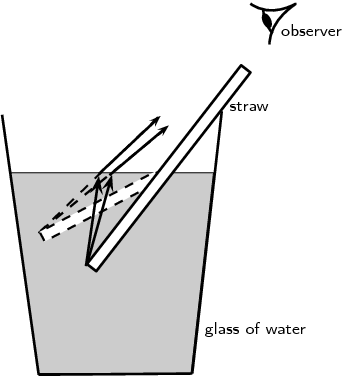Figure 5.8: Due to refraction, a straw in a glass of water appears bent when an observer looks down at an angle from above the water surface.
temp text

### Refractive index (ESBN5)

The speed of light and therefore the degree of bending of the light depends on the refractive index of material through which the light passes. The refractive index (symbol $$n$$) is the ratio of the speed of light in a vacuum to its speed in the material.

Refractive index

The refractive index (symbol $$n$$) of a material is the ratio of the speed of light in a vacuum to its speed in the material and gives an indication of how difficult it is for light to get through the material.

$n = \frac{c}{v}$ where $\begin{array}{rl} n &= \text{refractive index (no unit)} \\ c &= \text{speed of light in a vacuum } (\text{3,00} \times \text{10}^{\text{8}}\text{ m·s^{-1}}) \\ v &= \text{speed of light in a given medium } (\text{m·s^{-1}}) \\ \end{array}$

Using the definition of refractive index $n = \frac{c}{v}$ we can see how the speed of light changes in different media, because the speed of light in a vacuum, $$c$$, is constant.

If the refractive index, $$n$$, increases, the speed of light in the material, $$v$$, must decrease. Therefore light travels slower through materials of high refractive index, $$n$$.

temp text

Table 5.1 shows refractive indices for various materials. Light travels slower in any material than it does in a vacuum, so all values for $$n$$ are greater than $$\text{1}$$.

 Medium Refractive Index Vacuum $$\text{1}$$ Helium $$\text{1,000036}$$ Air* $$\text{1,0002926}$$ Carbon dioxide $$\text{1,00045}$$ Water: Ice $$\text{1,31}$$ Water: Liquid ($$\text{20}$$$$\text{°C}$$) $$\text{1,333}$$ Acetone $$\text{1,36}$$ Ethyl Alcohol (Ethanol) $$\text{1,36}$$ Sugar solution ($$\text{30}\%$$) $$\text{1,38}$$ Fused quartz $$\text{1,46}$$ Glycerine $$\text{1,4729}$$ Sugar solution ($$\text{80}\%$$) $$\text{1,49}$$ Rock salt $$\text{1,516}$$ Crown Glass $$\text{1,52}$$ Sodium chloride $$\text{1,54}$$ Polystyrene $$\text{1,55}$$ to $$\text{1,59}$$ Bromine $$\text{1,661}$$ Sapphire $$\text{1,77}$$ Glass (typical) $$\text{1,5}$$ to $$\text{1,9}$$ Cubic zirconia $$\text{2,15}$$ to $$\text{2,18}$$ Diamond $$\text{2,419}$$ Silicon $$\text{4,01}$$
Table 5.1: Refractive indices of some materials. $$n_{\text{air}}$$ is calculated at standard temperature and pressure (STP).

## Worked example 1: Refractive index

Calculate the speed of light through glycerine which has a refractive index of $$\text{1,4729}$$.

### Determine what is given and what is required

We are given the refractive index, $$n$$ of glycerine and we need to calculate the speed of light in glycerine.

### Determine how to approach the problem

We can use the definition of refractive index since the speed of light in vacuum is a constant and we know the value of glycerine's refractive index.

### Do the calculation

$n = \frac{c}{v}$ Rearrange the equation to solve for $$v$$ and substitute in the known values: \begin{align*} v &= \frac{c}{n} \\ &= \frac{\text{3} \times \text{10}^{\text{8}}\text{ m·s$^{-1}$}}{\text{1,4729}} \\ &= \text{2,04} \times \text{10}^{\text{8}}\text{ m·s$^{-1}$} \end{align*}

temp text

## Refractive index

Textbook Exercise 5.2

Use the values given in Table 5.1, and the definition of refractive index to calculate the speed of light in water (ice).

We look in Table 5.1 and find that the refractive index of water (ice) is $$\text{1,31}$$. So the speed of light in ice is:

\begin{align*} v &= \frac{c}{n} \\ &= \frac{\text{3} \times \text{10}^{\text{8}}\text{ m·s$^{-1}$}}{\text{1,31}} \\ &= \text{2,29} \times \text{10}^{\text{8}}\text{ m·s$^{-1}$} \end{align*}

Calculate the refractive index of an unknown substance where the speed of light through the substance is $$\text{1,974} \times \text{10}^{\text{8}}$$ $$\text{m·s^{-1}}$$. Round off your answer to 2 decimal places. Using Table 5.1, identify what the unknown substance is.

We are told that the speed of light in the unknown substance is: $$\text{1,974} \times \text{10}^{\text{8}}$$ $$\text{m·s^{-1}}$$. We use this to find the refractive index of the substance:

\begin{align*} v &= \frac{c}{n} \\ n &= \frac{c}{v} \\ &= \frac{\text{3} \times \text{10}^{\text{8}}\text{ m·s$^{-1}$}}{\text{1,974} \times \text{10}^{\text{8}}\text{ m·s$^{-1}$}} \\ &= \text{1,52} \end{align*}

We look on Table 5.1 for the substance that has this refractive index and find that the substance is crown glass.

### Optical density (ESBN6)

Optical density is a measure of the refracting power of a medium. In other words, the higher the optical density, the more the light will be refracted or slowed down as it moves through the medium. Optical density is related to refractive index in that materials with a high refractive index will also have a high optical density. Light will travel slower through a medium with a high refractive index and high optical density and faster through a medium which has a low refractive index and a low optical density.

Optical density

Optical density is a measure of the refracting power of a medium.

temp text

### Representing refraction with ray diagrams (ESBN7)

It is useful to draw ray diagrams to understand how the geometrical optics concepts we have discussed previously work. Before we can draw the diagrams we need to define a few concepts such as the normal to a surface, the angle of incidence and the angle of refraction.

Normal

The normal to a surface is the line which is perpendicular to the plane of the surface.

Angle of incidence

The angle of incidence is the angle defined between the normal to a surface and the incoming (incident) light ray.

Angle of refraction

The angle of refraction is the angle defined between the normal to a surface and the refracted light ray.Figure 5.9: The diagram shows the boundary between two media: water and air. An incoming light ray is refracted when it passes through the surface of the water into the air. The angle of incidence is $$\theta_{1}$$ and the angle of refraction is $$\theta_{2}$$.

When light travels from one medium to another, it is refracted. If the angle of incidence is not equal to zero, the light ray will change direction from its original path as it is refracted.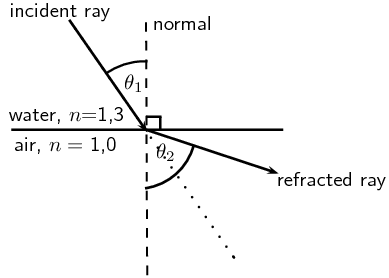Figure 5.10: Light is moving from an optically dense medium to an optically less dense medium.Figure 5.11: Light is moving from an optically less dense medium to an optically denser medium.
temp text

## Propagation of light from air into glass and back into air

### Aim

To investigate propagation of light from air into glass and back into air

### Apparatus

ray box, rectangular glass block, plain paper, pencil, ruler, protractor.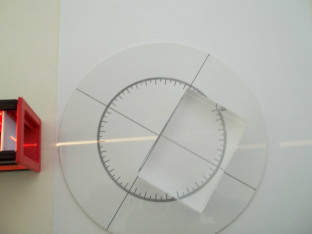### Method

1. Place the glass block on a plain piece of paper and use your pencil to draw around the block to make its outline on the paper.

2. Now turn on the ray box and aim the light ray through the left side of the glass block as illustrated in the diagram: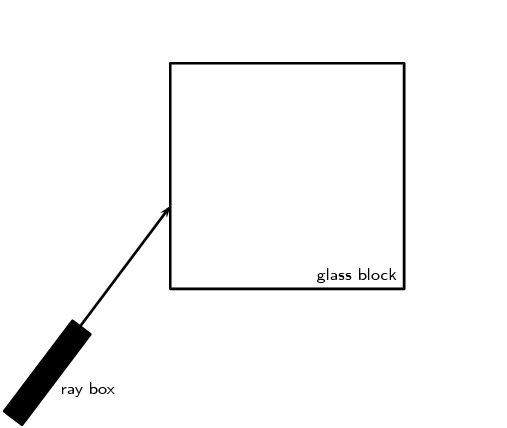3. Now use your pencil to draw a dot somewhere on the incident light ray and another dot at the point where it enters the glass block.

4. Use your pencil to draw a dot at the point where the light exits the glass block and also somewhere else along the exiting ray.

5. Turn off the ray box and remove the glass block from the paper. Use your ruler to join the dots so that you have drawn a picture that looks like the figure above.

6. Now draw the normals to the surfaces where the light ray enters and leaves the block and mark the angle of incidence and angle of refraction on the left surface and the right surface.

### Questions for discussion

1. At the surface where the light enters the glass block, what do you notice about the angle of incidence compared to the angle of refraction?

2. Now look at the surface where the light exits the glass block. Compare the angle of incidence and angle of refraction here.

3. How do the optical densities and indices of refraction for air and glass compare?

A recommended experiment for informal assessment is also included. This investigates the propagation of light from one medium into another. Learners will need glass blocks of various shapes, a transparent container, water, plain paper, a pencil, a ruler, a protractor and a ray box. The questions for discussion at the end of the experiment get learners thinking about what they found in the experiment.

## Propagation of light from one medium into another medium

### Aim

To investigate the propagation of light from one medium into another

### Apparatus

ray box, glass blocks of various shapes, a transparent container filled with water, plain paper, pencil, ruler, protractor.

### Method

Starting with the rectangular glass block, repeat the steps below for each of the various differently shaped glass blocks:

1. Place the glass block on a plain piece of paper and use your pencil to draw around the block to make its outline on the paper.

2. Turn on the ray box and aim the light ray through one of the block's surfaces.

3. Draw a dot at the point where the light enters the block and another dot somewhere else along the incident ray. Also draw a dot at the point where the ray exits the surface of the block and another somewhere along the exiting ray.

4. Remove the glass block and turn off the ray box. Use your ruler to join the dots.

5. Now draw the normals to the surfaces where the light ray enters and exits the block.

6. Use your protractor to measure the angles of incidence and refraction at the surfaces where the light ray enters and exits the block.

Now follow the same steps as before but place differently coloured filters at the surface of the blocks where the light enters the block.

Lastly, replace the glass blocks with the container of water and repeat the same steps as above. For this case, try to aim the ray box so that the angle of incidence on the water container is the same as it was for the rectangular glass block you investigated first. This is easier if you place your new piece of paper on top of the drawing you made with the rectangular block. Line up the water container where you drew the edge of your glass block. Then you can aim the ray box so that the light lines up with the incident ray on your paper and follow the steps as before.

### Questions for discussion

1. For each of your differently shaped glass blocks, how does the angle of incidence going from air to glass compare to the angle of refraction?

2. What happens when you place a coloured filter at the boundary between the air and a glass block?

3. When you compare your two diagrams for the rectangular glass block and the water container, if you did it correctly, the angles of incidence from air to glass/water should be the same. What can you say about the angles of refraction for glass and water respectively? How does this compare with what you already know about the refractive indices or optical densities of these materials?

## Refraction

Textbook Exercise 5.3

Explain refraction in terms of a change of wave speed in different media.

Refraction occurs at the boundary of two media when light travels from one medium into the other and its speed changes but its frequency remains the same. If the light ray hits the boundary at an angle which is not perpendicular to or parallel to the surface, then it will change direction and appear to ‘bend’.

We can also say that refraction is the bending of light caused by a change in the speed of light as it moves from medium to another.

In the diagram, label the following:

1. angle of incidence
2. angle of refraction
3. incident ray
4. refracted ray
5. normal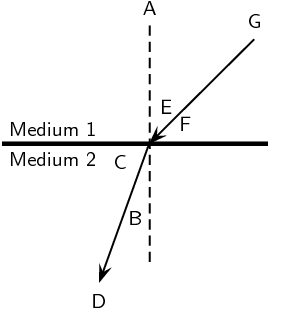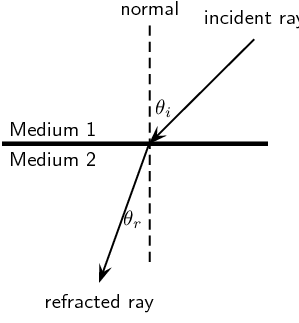What is the angle of refraction?

The angle of refraction is the angle defined between the normal to a surface and the refracted light ray.

Describe what is meant by the refractive index of a medium.

The refractive index (symbol n) of a material is the ratio of the speed of light in a vacuum to its speed in the material and gives an indication of how difficult it is for light to get through the material.

In the diagram, a ray of light strikes the interface between two media.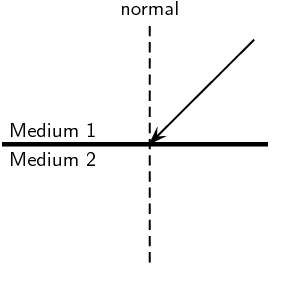Draw what the refracted ray would look like if:

medium 1 had a higher refractive index than medium 2.medium 1 had a lower refractive index than medium 2.Challenge question: What values of $$n$$ are physically impossible to achieve? Explain your answer. The values provide the limits of possible refractive indices.

A refractive index of less than 1 is impossible. This would mean that light in this medium was travelling at a speed faster than the speed of light in a vacuum. However scientists have produced materials with negative refractive indices. So the values of n that are impossible to achieve physically would be: $$0 \leqslant n < 1$$.

Challenge question: You have been given a glass beaker full of an unknown liquid. How would you identify what the liquid is? You have the following pieces of equipment available for the experiment: a laser or ray box, a protractor, a ruler, a pencil, and a reference guide containing optical properties of various liquids.

The answer to this question is covered in the experiment on using Snell's law to determine the refractive index of an unknown liquid.